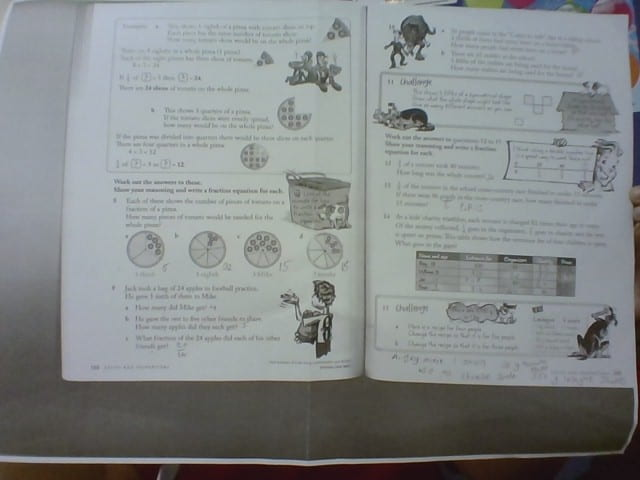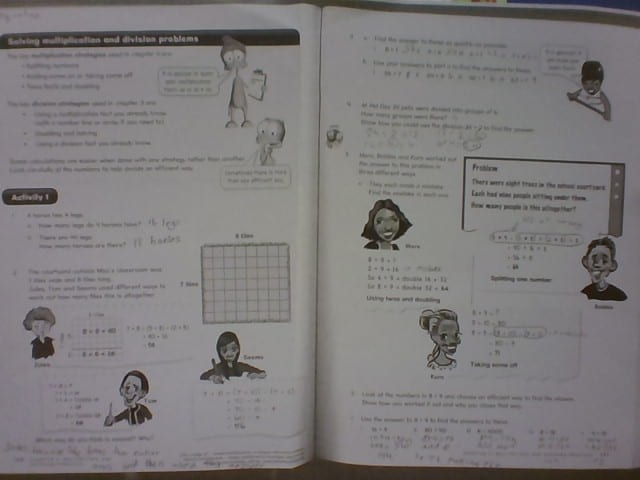# Tag: Maths

## How to Simplify an equivalent fractionToday for maths, at the end of our maths task we had to create a google draw, and pick a problem from the maths task we had recently done. We then had to explain how to solve them on the google draw step by step. Overall, this was quick and easy to do. I hope you learn something from this, or gain knowledge from this!

## Equivalent Fractions

Today for maths we are learning how to find simplify equivalent fractions,  we are also learning to write equivalent fractions down. I didn’t understand this at first but as I went through thoroughly, I had learnt a lot from it. In the end I found this task sort of challenging but relieved to do. I hope you enjoy!

## Mixed and Improper Fractions

Today for maths, we had to learn all about mixed fractions with Improper fractions. I found this difficult to do in the beginning but once I got used to it I managed to understand it.

## Fractions WorksheetToday for maths, we had to do our worksheets after we had finished the maths task. I have managed to completed this, this was about fractions and solving problems with it.

## Fractions Level 2

Today for maths, we had to learn about fractions, and how to add and subtract with the same denominator. I found this easy to do in the end, I now understand how to do these things successfully.

## BMF 3

Today for maths we had BMF for today’s task. BMF stands for Building Maths Fitness, this was kind of challenging for me to do but I have managed to complete this in the end.

## Fractions ExampleFor today’s maths we had to have an example of what a denominator and a numerator looked like, we also had to explain what these two things meant. This is my example of what 2 over 3 would look like. Overall this was pretty easy to do, I hope you enjoyed and learnt something from this.

## Maths Worksheet 2Today for maths, we had this worksheet we had to complete. I have done both sides of this worksheet and completed it, This was sort of challenging considering that we had to find the mistake in some equations.

## Building Maths Fitness

Today for maths, we had to do a warm up of Building Maths Fitness 2. This was challenging for me to do but in the end I have managed to complete this task.

## Worksheet DivisionThis is my worksheet for division, I have finished and completed. This was not as challenging but easy to do.